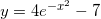# Find Range of Exponential Functions

Find the range of real valued exponential functions using different techniques. Matched problems are also included with their answers at the bottom of the page.

## Examples with Solutions

### Example 1

Find the Range of function f defined by#### Solution to Example 1

• Let us first write the above function as an equation as follows• solve the above function for x
-x + 2 = ln (y)
x = 2 - ln (y)
• x is a real number if y > 0 (argument of ln y must be positive). Hence the range of function f is given by
y > 0 or the interval (0 , +∞)
See graph of f below and examine the range graphically.#### Matched Problem 1:

Find the range of function f defined by### Example 2

Find the Range of function f defined by#### Solution to Example 2

• Write the given function as an equation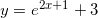• Solve the above equation for x
x = (1 / 2)(ln (y - 3) -1)
• x is a real number for y - 3 > 0 (argument of ln (y - 3) must be positive). The range of the given function is then given by
y > 3 or in interval form (3 , +∞)
See graph of f below and examine the range graphically.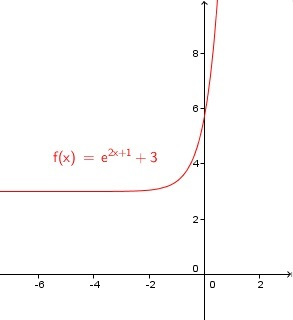#### Matched Problem 2:

Find the range of function f defined by### Example 3

Find the Range of function f defined by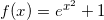#### Solution to Example 3

• Write the given function as an equation• Solve the above for x to obtain
x2 = ln(y - 1)
x = + or - √[ ln(y - 1) ]
• The above solutions are real if
ln(y - 1) ≥ 0
y - 1 ≥ 1
y ≥ 2
• Hence the range of the given function is given by
y ≥ 2 or in interval form [ 2 , + ∞ )

See graph of f below and examine the range graphically.#### Matched Problem 3:

Find the range of function f defined by### Example 4

Find the Range of function f defined by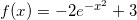#### Solution to Example 4

• We first write the given function as an equation as follows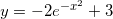• Solve the above for x
y - 3 = -2 e-x2
e-x2 = (y - 3) / (-2)
-x2 = ln [ (y - 3) / (-2) ]
x = + or - √( - ln [ (y - 3) / (-2) ])
• x is real if the argument of ln is positive and the radicand is positive or zero. Hence the following inequalities
(y - 3) / (-2) > 0 and - ln [ (y - 3) / (-2) ] ≥ 0
the solution set of (y - 3) / (-2) > 0 is y < 3
the solution set of - ln [ (y - 3) / (-2) ] ≥ 0 is given by (y - 3) / (-2) ≤ 1 which gives y ≥ 1
• the range of f is given by
1 ≤ y < 3 or in interval form [ 1 , 3 )

See graph of f below and examine the range graphically.#### Matched Problem 4:

Find the range of function f defined by